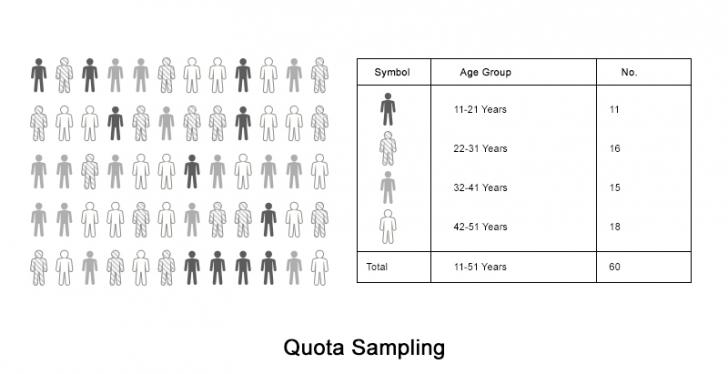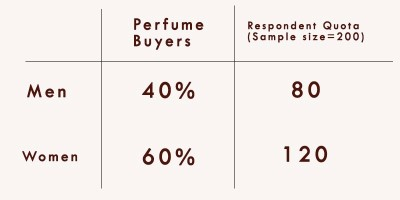# Quota Sampling

Sampling is the process of selecting a subset of the population for analysis to save time and resources. In some cases, the properties or preferences of the population is determined by certain characteristics that puts them in certain groups e.g. the preference of clothes depends on age is different for children, teenagers, college students and working professionals.

Quota sampling helps us in the selection of the samples by providing important metadata about how many samples need to be taken for each target group so that they are in proportion to the original population.

When we take the samples from a group of items with specific traits or specific characteristics in proportion to their size in the population, this is called quota sampling.

## Example of Quota Sampling

Suppose that you are required to conduct a survey regarding the choice of colors by females for a fashion designer. To get an accurate view of the data, you will need to collect data from different sections of the population of different age groups.

If you do this randomly, it can be a difficult task because the place where you can find the samples for each group is different. Teenagers hang out at completely different places than old people and their preferences are greatly shaped by their age as well. To make life easier, what you can do is divide the whole sample size into several fractions and assigning each of them to a section of the population.In the above figure, the population is distributed randomly. i.e., the women of different age are present. The assessment of the population needs to be done first. The next step becomes to calculate the number of samples that are to be taken from each group. Now that you know how many samples of each age group you need, you can plan your data collection better.

## Process of Quota Sampling

Quota Sampling is a technical job and requires some steps to be handled carefully. The steps are explained below:

### 1. Assess the Data and Determine the Traits to be chosen for Classification

In this step, we analyze our data and choose the traits or characteristics to be chosen for the classification of the data. Traits can be:

• Age Group
• Caste
• Height
• Level of Education
• Sex
• Level of Employment
• Country of Origin

### 2. Determine the number of Samples to be taken from each Group

In this step, we calculate the number of samples that are to be taken from each of the groups. This is done proportionally with the formula:

Number of Samples taken from a group

= Number of Items in the Group \$times\$ (Total number of Samples to be taken \$div\$ Total number of Items in the population)

### 3.Selection of Samples

In this step, we select the samples in proportion to the number of samples from each group as calculated in Step 2.As shown in the diagram above, we calculated the relative percentages of different groups of the population and calculated how many samples of each group we needed in the same proportion. Now that we know how many samples are needed, it is much easier to divide up the work of sample collection.

Quota Sampling is widely adopted these days because of its many benefits. Some of the advantages are stated below:

• Quota Sampling is an economic process. It saves time and money both at the same time by giving us important information about how many samples of each group we need to collect
• The extra information speeds up the sampling process
• We can get a high level of accuracy because quota sampling is scientific with a well defined process